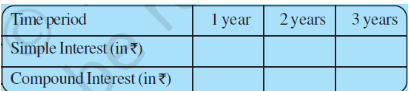# Q2. Principal =  Rs.1000, Rate = 8% per annum. Fill in the following table and find which type of interest (simple or compound) changes in direct proportion with time period.Given that Principal (P) = 1000 and Rate (r) = 8% per annum(per year).

Calculating the Simple Interest:

Formula for the simple interest is =

So, for 1 year:

.

for 2 years:

.

similarly for 3 years:

.

Calculating the Compound Interest :

The formula for the compound interest is

.

So for 1 year:

.

for 2 years:

.

similarly for 3 years:

.

Hence we haveIn case of simple interest

Simple interest is directly proportional with time.

While in case of compound interest:

does not give the same constant.

Compound interest is not directly proportional with time.

## Related Chapters

### Preparation Products

##### JEE Main Rank Booster 2021

This course will help student to be better prepared and study in the right direction for JEE Main..

₹ 13999/- ₹ 9999/-
##### Rank Booster NEET 2021

This course will help student to be better prepared and study in the right direction for NEET..

₹ 13999/- ₹ 9999/-
##### Knockout JEE Main April 2021 (Easy Installments)

An exhaustive E-learning program for the complete preparation of JEE Main..

₹ 4999/-
##### Knockout NEET May 2021

An exhaustive E-learning program for the complete preparation of NEET..

₹ 22999/- ₹ 14999/-